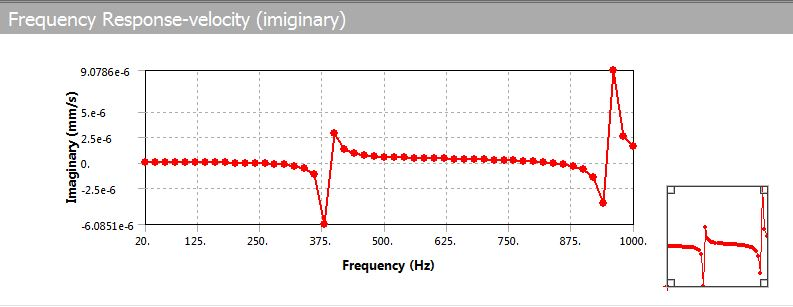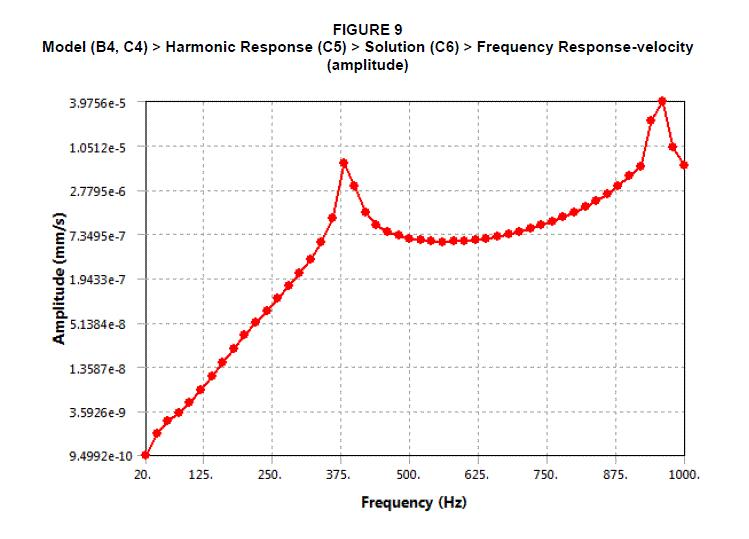# converting to RMS value from Harmonic response spectrum

Good Day,

Dear Guys,

I had a problem to converting spectrum data to Rms Value, i did not want the negative value to be appeared , how can i do it?• edited March 2019

I am not sure if I understand your question. RMS means root mean squared. You will square the value, so eventually everything is positive under square root. https://en.wikipedia.org/wiki/Root_mean_square

• edited March 2019

RMS is more for summing stresses in response spectrum analysis (PSD or response spectra/earthquake say).

I think you mean amplitude.

The FRF is a complex function/number, and you are showing the imaginary part of that complex number.

Complex numbers and FRFS can be represented thus by a phase and an amplitude (positive number). Thus for complex number z:

z = x + i*y = ¦z¦*exp(i*phase),

https://en.wikipedia.org/wiki/Complex_number

So you need/mean the amplitude of the FRF which is:

¦z¦=sqrt(x^2+y^2)

You can set amplitude under details, options, and display in the frequency response.

Search the ansys help manual for:

### Frequency Response and Phase Response

• edited March 2019

Thank you for your help sir, i had change to amplitude, are you sure it indicate the RMS value, since its look too different between the imiginary result before

.• how can @Hadi96 ,you get a amplitude graph ?

have to apply the rotating force and fixed rotation